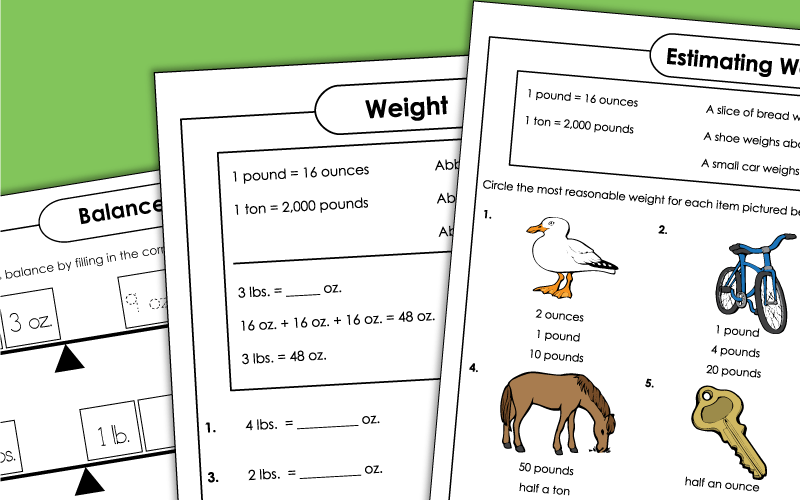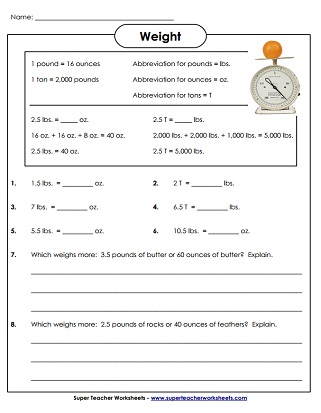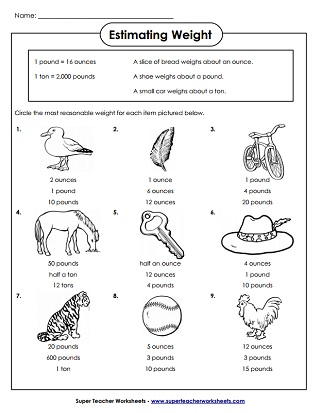# Measurement Worksheets: Weight (Lbs. and Oz.)

These printable measurement worksheets will help students: measure the weight of objects in standard or customary units (pounds, ounces, and tons), estimate the weight of objects, and convert weights from pounds to ounces.Choose the best weight estimate (lbs., oz., and tons) for each of the nine pictures shown.
Convert to and from tons and pounds and ounces.  Includes half units.
A set of word problems that require students to convert weights from oz to lbs and lbs to oz.
A more challenging set of word problems related to measuring weight.
Determine the correct weight amounts (lbs. and oz.) to make the scales balance.

## Also on Super Teacher Worksheets...

Capacity (Gallons, Quarts, etc.)

Measure capacity/volume using cups, quarts, pints, and gallons

Linear Measurement (Centimeters, Millimeters)

Measure to the nearest millimeter or centimeter; Metric measurement printables

## Worksheet ImagesMy Account
Site Information